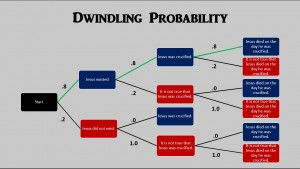# In Defense of Dwindling Probability

One claim involved in the case for the resurrection of Jesus is this:
D.  Jesus died on the same day he was crucified.
The truth of this claim depends on the truth of some prior claims:
E.  Jesus existed.
C. Jesus was crucified.
A probability tree diagram can illustrate how claim (D) involves dwindling probability (for a better view, click on the image):There is ONLY ONE PATH that results in a probability greater than ZERO for claim (D).  I will not argue for the correctness or accuracy of the probability estimates used in the diagram.  These numbers are for the purpose of illustration, to show the way dwindling probabilty works.
Let’s say that our basic stock of historical facts is f.  These facts would include the contents of the canonical Gospels, plus “outsider” sources, plus “insider” non-narrative sources, plus non-canonical Gospels/narrative sources related to Jesus.
The first green branch indicates that the probability that Jesus existed, given our basic stock of historical facts is .8 :
P(E/f) = .8
The second green branch indicates that the probability that Jesus was crucified, given our basic stock of historical facts PLUS the existence of Jesus is .8:
P(C/f & E) = .8
The third green branch indicates that the probability that Jesus died on the same day he was crucified, given our basic stock of historical facts PLUS the existence of Jesus PLUS the crucifixion of Jesus is .8:
P(D/f & E & C) = .8
The probability that Jesus died on the same day he was crucified given our stock of historical facts is equal to:
the probability of Jesus existing given our historical facts TIMES the probability of Jesus being crucified given our historical facts and the existence of Jesus TIMES the probability of Jesus dying on the same day he was crucified given our historical facts and the existence of Jesus and the crucifixion of Jesus.
P(D/f)P(E/f) x P(C/f & E) x P(D/f & E & C)
Based on the probability estimates in the above diagram, we can fill in the numbers:
P(D/f) = .8 x .8 x .8 = .512  or approximately .5
Although at each branch the probability was high (.8), multiplying the three probabilities together reduces the probability of claim (D) to about .5 which is NOT a high probability.